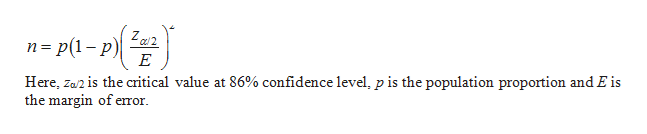# If subject 101is 71 years old. Divide the age by 10 and put a % on it, that is your desire margin of error in percentage points. Find the sample size required to develop an 86% confidence interval on a population proportion that will result in that margin error.

Question
1 views

If subject 101is 71 years old. Divide the age by 10 and put a % on it, that is your desire margin of error in percentage points. Find the sample size required to develop an 86% confidence interval on a population proportion that will result in that margin error.

check_circle

Step 1

Solution:

The formula for the sample siz...help_outlineImage TranscriptioncloseZa2 n= p(1- p) Here, za2 is the critical value at 86% confidence level, p is the population proportion and E is the margin of error. fullscreen

### Want to see the full answer?

See Solution

#### Want to see this answer and more?

Solutions are written by subject experts who are available 24/7. Questions are typically answered within 1 hour.*

See Solution
*Response times may vary by subject and question.
Tagged in

### Other